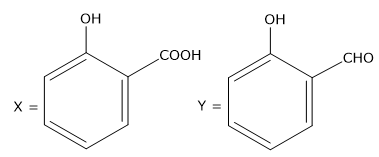Request a call back

## CBSE Class 12 Chemistry Previous Year Question Paper Term 1 2021 SSK-3-Set-4

Your Class 12 Science syllabus for Chemistry is designed to make you competent for pursuing higher education in basic sciences, medicine, engineering, etc.  You’ll learn about chemistry concepts such as biomolecules, redox reactions, s-Block elements, thermodynamics, and more. On TopperLearning, you’ll get access to several study resources for learning these concepts well.

Browse through our e-learning resources like sample papers, video lessons, previous year papers, etc. Our video lessons are presented by Chemistry experts to help you enjoy learning Chemistry topics such as properties of colloids, coordination compounds, aldehydes and ketones, DNA, polymers, etc.

Practise likely Chemistry exam questions by using our CBSE Class 12 Science sample papers with solutions and previous paper with solutions. Use our NCERT Chemistry Class 12 solutions for chapter-wise revision. Our MIQs are also beneficial for quick revision of important Chemistry concepts. Study Chemistry concepts and practise our expert solutions for a stress-free Chemistry board exams and score more marks.

CBSE Class 12 Chemistry introduces you to a number of laws, reactions, elements, and other concepts. When you sit to study, you may get confused with the various concepts. The best way to revise Chemistry would be to practise the solutions for Chemistry questions that’s prepared by experts.

On the TopperLearning portal, you’ll find expert solutions to NCERT textbook questions, sample paper questions and questions from previous year CBSE Class 12 Chemistry exam papers. Our Chemistry experts have presented the solutions in a step-wise format to help you understand the solutions. Also, concept notes are added for certain solutions to give you more details about the concept used for answering a question.

NCERT Chemistry Class 12 solutions are important for you to practise different types of questions from your Chemistry textbook. Whether it is a short answer question or a long answer question, you’ll be ready to answer questions accurately in your exam with sufficient practise of the Chemistry solutions.

SECTION A

This section consists of 25 multiple choice questions with overall choice to attempt any 20 questions. In caw more than desirable number of questions are attempted. ONLY first 20 will be considered for evaluation.

Q 1. Which one of the following pairs will form an ideal solutions?

1. Chloroform and acetone
2. Ethanol and acetone
3. n-hexane and n-heptane
4. Phenol and aniline

Q 2.Which of the following is known as amorphous solid?

1. Glass
2. Plastic
3. Rubber
4. All of the above

Q 3.The structure of pyrosulphuric acid is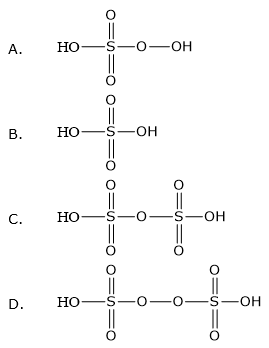Q 4.The C-O-H bond angle in alcohol is

1. Slightly greater than 109°28’.
2. Slightly less than 109°28’.
3. Slightly greater than 120°
4. Slightly less than 120°

Q 5.Consider the following reaction: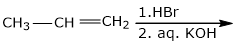The major end product is

1.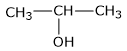2.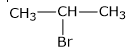3. CH3-CH2-CH2-OH
4. CH3-CH2-CH2-Br

Q 6.Nucleosides are composed of

1. a pentose sugar and phosphoric acid
2. a nitrogenous base and phosphoric acid
3. a nitrogenous base and a pentose sugar
4. a nitrogenous base, a pentose sugar and phosphoric acid

Q 7.The oxidation state of -2 is most stable in:

1. O
2. S
3. Se
4. Te

Q 8.Which of the following is not a characteristic of a crystalline solid?

1. A true solid
2. A regular arrangement of constituent particles
3. Sharp melting point
4. Isotropic in nature

Q 9.Which of the following formula represents Raoult’s law for a solution containing non-volatile solute?

1. Psolute = Posoluse  - Xsolute
2. P = KH.X
3. PTotal = Psolvent
4. Psolute = Posolvent - XSolvent

Q 10. An azeotropic solution of two liquids has a boiling point lower than either of the two when it

1. Shows a positive deviation from Raoult’s law.
2. Shows a negative deviation from Raoult’s law.
3. Shows no deviation from Raoult’s law.
4. is saturated.

Q 11. Which of the following crystal will show metal excess defect due to extra cation?

1. AgCl
2. NaCl
3. FeO
4. ZnO

Q 12. Which of the following acids reacts with acetic anhydride to from a compound Aspirin?

1. Benzoic acid
2. Salicylic acid
3. Phthalic acid
4. Acetic acid

Q 13. Which of the following statement is wrong?

1. Oxygen shows pπ – pπ bonding.
2. Sulphur shows little tendency of catenation.
3. Oxygen is diatomic whereas Sulphur is polyatomic.
4. O-O bond is stronger than S-S bond.

Q 14. Amino acids which cannot be synthesized in the body and must be obtained through diet are known as

1. Acidic amino acids
2. Essential amino acids
3. Basic amino acids
4. Non-essential amino acids

Q 15. Which one of the following halides contains Csp2 – X bond?

1. Allyl halide
2. Alkyl halide
3. Benzyl halide
4. Vinyl halide

Q 16. On mixing 20 mL of acetone with 30 mL of chloroform, the total volume of the solution is

1. < 50 mL
2. = 50 mL
3. > 50 mL
4. = 10 mL

Q 17. Consider the following compounds: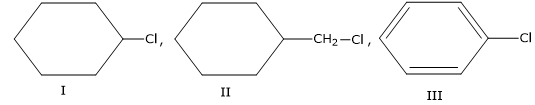the correct order of reactivity towards SN2 reaction
1. I > III > II
2. II > III > I
3. II > I > III
4. III > I > II

Q 18. Which of the following forms strong pπ – pπ bonding?
1. S8
2. Se8
3. Te8
4. O2

Q 19. F2 acts as a strong oxidising agent due to
1. Low Δbond H° and low Δhyd
2. Low Δbond H° and low Δhyd
3. High Δbond H° and high Δeg
4. Low Δhyd H° and low Δeg

Q 20. Which of the following sugar is known as dextrose?
1. Glucose
2. Fructose
3. Ribose
4. Sucrose

Q 21. Cu reacts with dilute HNO3 to evolve which gas?
1. N2O
2. NO2
3. NO
4. N2

Q 22. Which of the following is a network solid?
1. SO2
2. SiO2
3. CO2
4. H2O

Q 23. Major product formed in the following reaction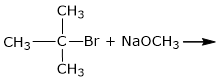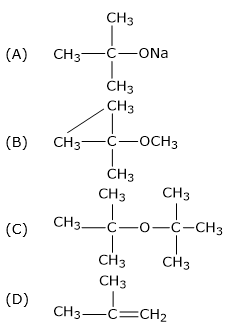Q 24. Chlorine reacts with cold and dilute NaOH to give
1. NaCl and NaClO3
2. NaCl and NaClO
3. NaCl and NaClO4
4. NaClO and NaClO3

Q 25. Elevation of boiling point is inversely proportional to
1. Molal elevation constant (Kb)
2. Molality (m)
3. Molar mass of solute (M)
4. Weight of solute (w)

SECTION B

This section consist of 24 multiple choice question with overall choice to attempt any 20 questions, In case more than desirable number of questions are attempted, ONLY first 20 will be considered for evaluation.

Q 26. An unknown gas ‘X’ is dissolved in water at 2:5 bar pressure and has mole fraction 0.04 is solution. The mole fraction of ‘X’ gas when the pressure of gas is doubled at the same temperature is
1. 0.08
2. 0.04
3. 0.02
4. 0.92

Q 27. The base which is present in DNA but not in RNA, is
1. Cytosine
2. Guanine
4. Thymine

Q 28. In the following reaction
The product formed is
1. CH3-CHO and CH3CH2OH
2. CH3-CH= CH- COOH
3. CH3 – CH= CH-CHO
4. CH3-CH2-CH2-CHO

Q 29. Enantiomers differ only in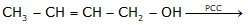1. Boiling point
2. Rotation of polarised light
3. Melting point
4. Solubility

Q 30. The number of lone pairs of electrons in XeF4 is
1. Zero
2. One
3. Two
4. Three

Q 31. Sulphuric acid is used to prepare more volatile acids from their corresponding salts due to its
1. Strong acidic nature
2. Low volatility
3. Strong affinity for water
4. Ability to act as a dehydrating agent

Q 32. An element with density 6 g cm-3 forms a fee lattice with edge length of 4 × 10-8 cm. The molar mass of the element is (NA = 6 ×1023 mol-1)
1. 57.6 g mol-1
2. 28.8 g mol-1
3. 82.6 g mol-1
4. 62 g mol-1

Q 33. In the reaction Compound ‘Y’ is-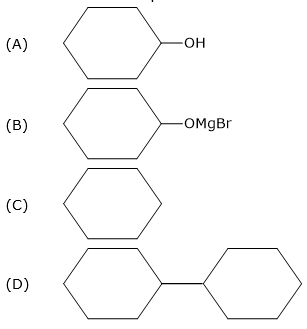Q 34. Which of the following is the weakest reducing agent in group 15?
1. NH3
2. PH3
3. AsH3
4. BiH3

Q 35. The boiling point of a 0.2 m solution of a non-electrolyte in water is (Ks for water = 0.52 K kg mol-1)
1. 100 °C
2. 100.52 °C
3. 100.104 °C
4. 100.26 °C

Q 36. Nucleic acids are polymer of
1. Amino acids
2. Nucleosides
3. Nucleotides
4. glucose

Q 37. Which of the following gas dimerises to become stable?
1. CO2 (g)
2. NO2 (g)
3. SO2 (g)
4. N2O (g)

Q 38. In the following diagram point, 'X' represents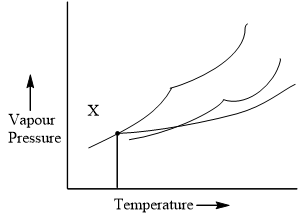1. Boiling point of solution
2. Freezing point of solvent
3. Boiling point of solvent
4. Freezing point of solution

Q 39. XeF6 on reaction with NaF gives
1. Na+[XeF7]-
2. [NaF2]-[XeF5]+
3. Na+[XeF6]-
4. [NaF2]+[XeF5]-

Q 40. Glucose on reaction with Br2 water gives:
1. Saccharic acid
2. Hexanoic acid
3. Gluconic acid
4. Salicylic acid

Q 41. Which of the following is optically inactive?
1. (+)- Butan-2-ol
2. (-) - Butan-2-ol
3. (±) - Butan-2-ol
4. (+) -2 - Bromobutane

Q 42. Which of the following is not a correct statement?
1. Halogens are strong oxidising agents
2. Halogens are more reactive than interhalogens.
3. All halogens arc coloured.
4. Halogens have maximum negative electron gain enthalpy.

Q 43. Which of the following has highest boiling point?
1. C2H5-F
2. C2H5-Cl
3. C2H5-Br
4. C2H5-l

Q 44. Which of the following isomer of pentane (C5H12) will give three isomeric monochlorides on photochemical chlorination?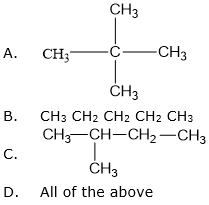Given below are the questions (45-49) labelled as Assertion (A) and Reason (R). Select the most appropriate answer from the options given below:

Q 37.   Assertion (A): A raw mango placed in a saline solution loses water and shrivel into pickle.

Reason (R): Through the process of reverse osmosis, raw mango shrivel into pickle.

A.       Both A and R arc true and R is the correct explanation of A.

B.       Both A and R arc true but R is not the correct explanation of A.

C.       A is true but R is false.

D.       A is false but R is true.

Q 38.   Assertion (A): H2S is less acidic than H2Te.

Reason (R): H-S bond has more bond Ho than H-Te bond

(A)    Both A and R arc true and R is the correct explanation of A

(B)    Both A and R arc true but R is not the correct explanation of A.

(C)    A is true but R is false.

(D)    A is false but R is true.

Q 39.   Assertion (A): Chlorobenzene is less reactive towards nucleophilic substitution reaction.

Reason (R): Nitro group in chlorobenzene increases its reactivity towards nucleophilic substitution reaction.

A.       Both A and R are true and R. is the correct explanation of A.

B.       Both A and R arc true but R is not the correct explanation of A.

C.       A is true but R is false.

D.       A is false but R is true.

Q 40.   Assertion (A): Due to Schottky defect, there is no effect on the density of a solid.

Reason (R): Equal number of cations and anions arc missing from their normal sites in Schottky defect.

(A)    Both A and R are true and R is the correct explanation of A.

(B)    Both A and R are true but R is not the correct explanation of A.

(C)    A is true but R is false.

(D)    A is false but R is true.

Q 41.   Assertion (A): Fluorine forms only one oxoacid HOF.

Reason (R): Fluorine atom is highly electronegative.

A.       Both A and R arc true and R is the correct explanation of A.

B.       Both A and R are true but K is not the correct explanation of A.

C.       A n true but K is false.

D.       A is false but R is true.

SECTION C

This section consists of 6 multiple choice questions with an overall choice to attempt any 5. In case more than desirable number of questions are attempted, ONLY first 5 will be considered for evaluation.

Q 42.   Match the following:

 I II (i)    Stoichiometric defects (A)   Crystalline solids (ii)   long range order (b)   F-centres (iii)  ….ABC ABC ABC……. (c)   Schottky and Frenkel defects (iv)Number of atoms per unit cell=2 (d)   fee structure (v)   Metal excess defect due to anionic vacancies

Which of the following is the best matched options?

(A)    (i) - (d), (ii) - (a), (iii) - (b), (iv) - (c)

(B)    (i) - (c), (ii) - (a), (iii) - (d), (v) - (b)

(C)    (i) - (c), (ii) - (a), (iii) - (d), (iv) - (b)

(D)    (i) - (a), (ii) - (b), (v) - (c), (iv) - (d)

Q 43.   Which of the following analogies is correct?

A.       XeF2 : linear :: XeF6 square planar

B.       moist SO2 : Reducing agent :: Cl2 : bleaching agent

C.       N2 : Highly reactive gas :: F2 : inert at room temperature

D.       NH3 : strong base :: HI : weak acid

Q 44.   Complete the following analogy :

Curdling of milk : A :: Alpha-helix : B

(A)    A : Primary structure              B : Secondary structure

(B)    A : Denatured protein            B : Primary structure

(C)    A : Secondary structure         B : Denatured protein

(D)    A : Denatured protein            B : Secondary structure

Given below are the questions (45-49) labelled as Assertion (A) and Reason (R). Select the most appropriate answer from the options given below:

Q 45. Assertion (A): A raw mango placed in a saline solution loses water and shrivel into pickle.

Reason (R): Through the process of reverse osmosis, raw mango shrivel into pickle.

1. Both A and R arc true and R is the correct explanation of A.
2. Both A and R arc true but R is not the correct explanation of A.
3. A is true but R is false.
4. A is false but R is true.

Q 46. Assertion (A): H2S is less acidic than H2Te.

Reason (R): H-S bond has more ∆bond Ho than H-Te bond

1. Both A and R arc true and R is the correct explanation of A
2. Both A and R arc true but R is not the correct explanation of A.
3. A is true but R is false.
4. A is false but R is true.

Q 47. Assertion (A): Chlorobenzene is less reactive towards nucleophilic substitution reaction.

Reason (R): Nitro group in chlorobenzene increases its reactivity towards nucleophilic substitution reaction.

1. Both A and R are true and R. is the correct explanation of A.
2. Both A and R arc true but R is not the correct explanation of A.
3. A is true but R is false.
4. A is false but R is true.

Q 48. Assertion (A): Due to Schottky defect, there is no effect on the density of a solid.

Reason (R): Equal number of cations and anions arc missing from their normal sites in Schottky defect.

1. Both A and R are true and R is the correct explanation of A.
2. Both A and R are true but R is not the correct explanation of A.
3. A is true but R is false.
4. A is false but R is true.

Q 49. Assertion (A): Fluorine forms only one oxoacid HOF.

Reason (R): Fluorine atom is highly electronegative.

1. Both A and R arc true and R is the correct explanation of A.
2. Both A and R are true but K is not the correct explanation of A.
3. A n true but K is false.
4. A is false but R is true.

SECTION C

This section consists of 6 multiple choice questions with an overall choice to attempt any 5. In case more than desirable number of questions are attempted, ONLY first 5 will be considered for evaluation.

Q 50. Match the following: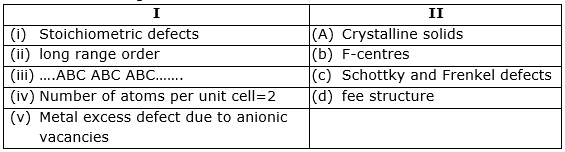Which of the following is the best matched options?

1. (i) - (d), (ii) - (a), (iii) - (b), (iv) - (c)
2. (i) - (c), (ii) - (a), (iii) - (d), (v) - (b)
3. (i) - (c), (ii) - (a), (iii) - (d), (iv) - (b)
4. (i) - (a), (ii) - (b), (v) - (c), (iv) - (d)

Q 51. Which of the following analogies is correct?

1. XeF2 : linear :: XeF6 square planar
2. moist SO2 : Reducing agent :: Cl2 : bleaching agent
3. C.N2 : Highly reactive gas :: F2 : inert at room temperature
4. D.NH3 : strong base :: HI : weak acid

Q 52. Complete the following analogy :

Curdling of milk : A :: Alpha-helix : B

1. A : Primary structure B : Secondary structure
2. A : Denatured protein B : Primary structure
3. A : Secondary structure B : Denatured protein
4. A : Denatured protein B : Secondary structure

Case : Read the passage given below and answer the following questions (53-55).

Alcohols and Phenols are acidic in nature. Electron withdrawing groups in phenol increase its acidic strength and electron donating groups decrease it. Alcohols undergo nucleophilic substitution with hydrogen halides to give alkyl halides. On oxidation primary alcohols yield aldehydes with mild oxidising agents and carboxylic acids with strong oxidising agents while secondary alcohols yield ketones. The presence of —OH groups in phenols activates the ring towards electrophilic substitution. Various important products are obtained from phenol like salicylaldehyde, salicylic acid, picric acid etc.

Q 53. Which of the following alcohols is resistant to oxidation?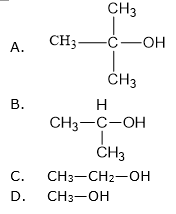Q 54. Which of the following group increases the acidic character of phenol?

1. CH3O-
2. CH3-
3. NO2-
4. All of these

Q 55 .Consider the following reaction: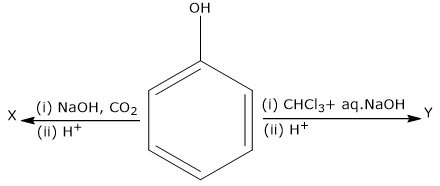the products X and Y are

 A.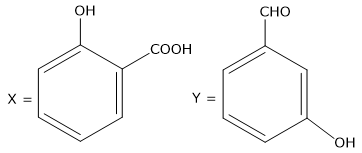B.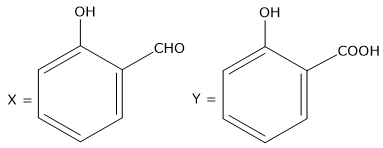C.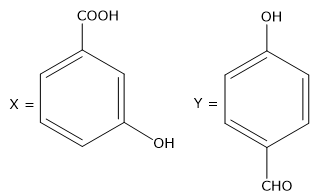D.